PCB inductors - 3.5 out of 5 based on 4 votes
Rating 3.50 (4 Votes)

PCB inductors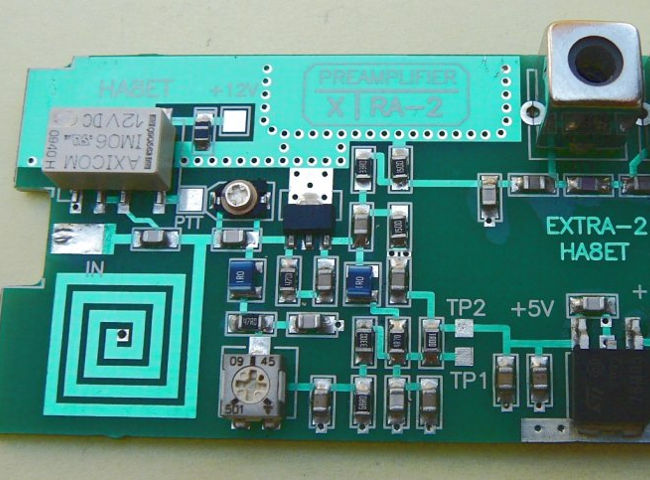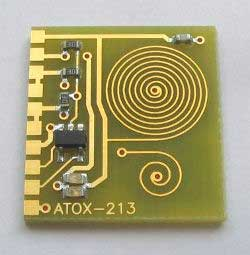Flat PCB coils are most often used in the VHF and UHF bands to reduce the size of the device. Usually, they are produced with round, square or meander form of turns, although it can be in the form of a polygon. With the advent technology of multilayer PCB, the multilayer coil appears. The use of a magnetic core is ineffective because such a core is far away from the turns of the coil and can change its inductance by 3 - 5 %, which in most cases is not enough. Therefore, printed inductors are used in most cases when the adjustment is not required and the value of the inductance units does not exceed microhenry. Q-factor of PCB inductors is generally lowered then Q-factor of the inductors having a coil of wire and less than that of the cylindrical microstrip coils. The self-capacitance of the printed inductors depends on the width of the spiral turns, the gap between them and the PCB material and can reach 3 to 5 pF, which is pretty high at such frequencies. All I have said is not in favor of flat PCB coils, however, the compact design along with the use of SMD components often plays a decisive role in use such inductors.

You can use the online calculator to calculate the PCB coils on our site.To compute inductance of PCB inductors we can use three pretty accurate methods.

1.The first approximation is based on a modification of an expression developed by H.A.Wheeler for the planar spiral inductors:• L- inductance (nH);
• µ0 = 4π*10-7 - magnetic constant;
• N - number of turns;
• Davg - average diameter of coil (µm) (expression);
• φ - fill factor (expression);
• The coefficients K1 and K2 are layout dependent and are shown in the following table:
Table I
LayoutK1K2
Square 2.34 2.75
Hexagonal 2.33 3.82
Octagonal 2.25 3.55

2.The second is a monomial expression derived from fitting to a large database of inductors (and the exact inductance values):where:

• L- inductance (nH);
• D - Outer diameter of the coil (µm);
• W - width of the PCB trace (µm);
• Davg - average diameter of coil (µm) (expression);
• N - number of turns;
• g = s - W  gap between turns (µm);
• And the coefficients β and αi are layout dependent and given in the following table:
Table II
Layoutβα1α2α3α4α5
Square  1.62·10-3  -1.21  -0.147  2.40  1.78  -0.03
Hexagonal  1.28·10-3  -1.24  -0.174  2.47  1.77 -0.049
Octagonal  1.33·10-3  -1.12  -0.163  2.43  1.75  -0.049

This formula is used in Coil32 for square PCB coil calculation.

3.The third is derived from electromagnetic principles by approximating the sides of the spirals as current-sheets:where

• L- inductance (nH);
• µ0 = 4π*10-7 - magnetic constant;
• N - number of turns;
• Davg - average diameter of coil (µm) (expression);
• φ - fill factor (expression);
• ci - factors from Table III (layout depending):
Table III
Layoutc1c2c3c4
Square   1.27  2.07  0.18  0.13
Hexagonal  1.09  2.23  0.00  0.17
Octagonal  1.07  2.29  0.00  0.19
Circle  1.00  2.46 0.00  0.20

For PCB inductor with round turns Coil32 uses the third method. The rearranged formula  is shown as Although the accuracy of this expression worsens as the ratio s/W becomes large, it exhibits a maximum error of 8% for s ≈ 3W. Note that typical practical integrated spiral inductors are built with w ≤ s. For highest Q-factor the optimum ratio d/D = 0.4 for circle coil and optimum d/D = 0.362 for square coil. The program automatically set inner diameter value for this ratio. In the calculation, we define the outer diameter of the coil and its layout. If the result of the calculation obtained unacceptable sizes of the printed trace, the outer diameter we can modify and recalculate the coil again.

All three expressions are accurate, with typical errors of 2–3%, and very simple, and are therefore excellent candidates for use in the design and synthesis PCB inductors. In all expressions, Davg factor is average diameter of the coil and φ is the filling factor that represents how hollow the inductor is. These factors are defined as: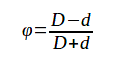• Davg - average diameter of coil;
• φ - fill factor;
• D - outer diameter of a coil;
• d - inner diameter of a coil;

Inductor as a straight microstrip calculates by the following expression: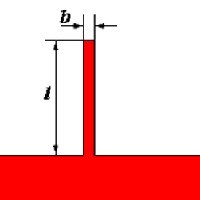• L - inductance (μH)
• l -trace length (mm)
• b - trace width (mm)

Such inductive elements are often used in filters UHF band. Because of what the self-capacitance of such inductive element is quite large, it is necessary to keep in mind that the more correct to represent it in the form of a segment of a transmission line with distributed parameters. However, for approximate calculations, this model is quite acceptable if this element works at frequencies much lower the self-resonance.

Plugin meandr_PCB allows calculating PCB inductors with meander layout of turns.

References:

Сomments from anonymous guests are enabled with moderation.

Coil Winding Machine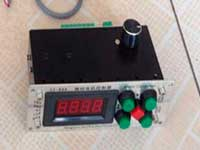US \$187.53
The system consists of individual components including a controller, a stepping motor, a foot switch, power adapter and output shaft. This design makes it very easy for you to integrate the system into your own project.

LC Meter LC200A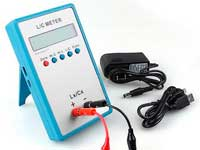US \$37.99
Measuring Capacitance Range:
0.01 pF - 100mF
Measuring Inductance Range:
0.001 uH - 100H
Measurement accuracy:1%.

RF Inductors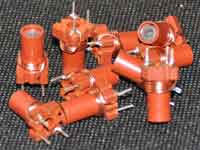US \$6.43
Variable Ferrite Core Inductor coil set 3.5Turns 25-100MHZ

Auto Ranging LCR Meter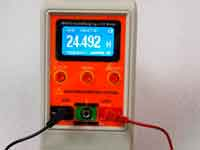US \$63.07
Meter can measure 0.00pF to 100.00mF capacitance, 0.000uH to 100.00H inductance and 0.00? to 10.000M? resistance. Very suitable for small value capacitor and inductor measurement.